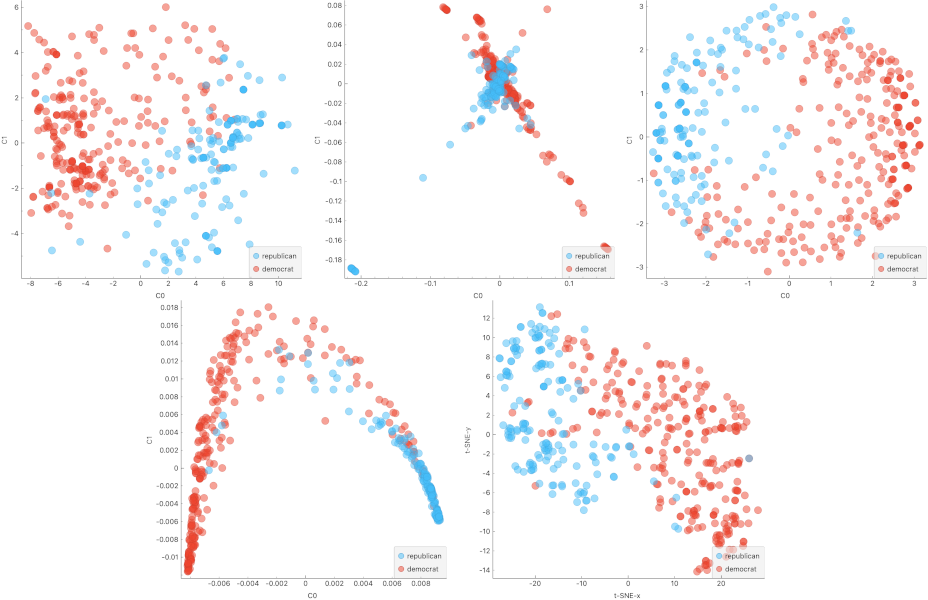# Manifold Learning¶

Nonlinear dimensionality reduction.

Inputs

• Data: input dataset

Outputs

• Transformed Data: dataset with reduced coordinates

Manifold Learning is a technique which finds a non-linear manifold within the higher-dimensional space. The widget then outputs new coordinates which correspond to a two-dimensional space. Such data can be later visualized with Scatter Plot or other visualization widgets.1. Method for manifold learning:

2. Set parameters for the method:

• t-SNE (distance measures):

• Euclidean distance

• Manhattan

• Chebyshev

• Jaccard

• Mahalanobis

• Cosine

• MDS (iterations and initialization):

• max iterations: maximum number of optimization interactions

• initialization: method for initialization of the algorithm (PCA or random)

• Isomap:

• number of neighbors

• Locally Linear Embedding:

• method:

• number of neighbors

• max iterations

• Spectral Embedding:

• affinity:

• nearest neighbors

• RFB kernel

3. Output: the number of reduced features (components).

4. If Apply automatically is ticked, changes will be propagated automatically. Alternatively, click Apply.

5. Produce a report.

Manifold Learning widget produces different embeddings for high-dimensional data.From left to right, top to bottom: t-SNE, MDS, Isomap, Locally Linear Embedding and Spectral Embedding.

## Preprocessing¶

All projections use default preprocessing if necessary. It is executed in the following order:

• continuization of categorical variables (with one feature per value)

• imputation of missing values with mean values

To override default preprocessing, preprocess the data beforehand with Preprocess widget.

## Example¶

Manifold Learning widget transforms high-dimensional data into a lower dimensional approximation. This makes it great for visualizing datasets with many features. We used voting.tab to map 16-dimensional data onto a 2D graph. Then we used Scatter Plot to plot the embeddings.